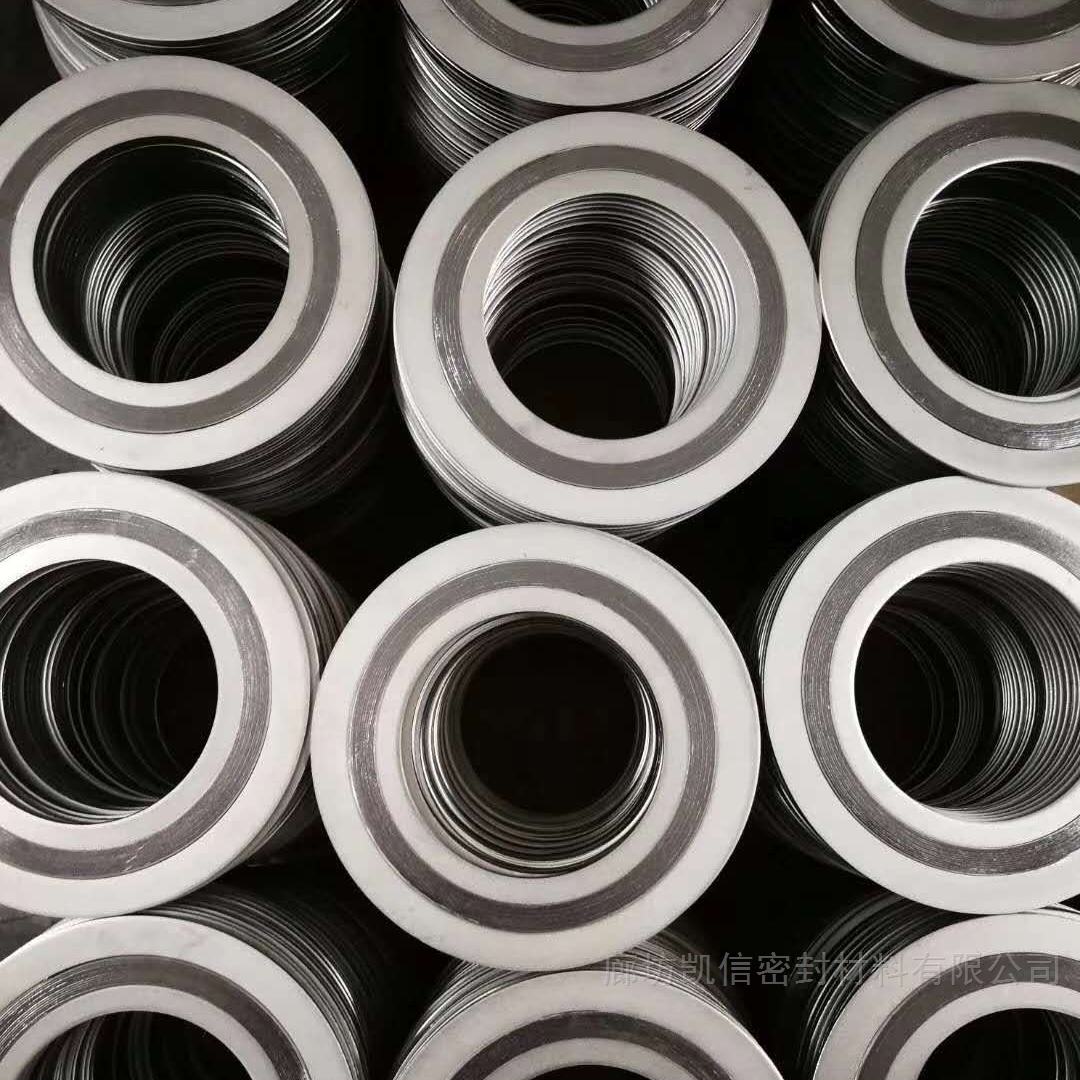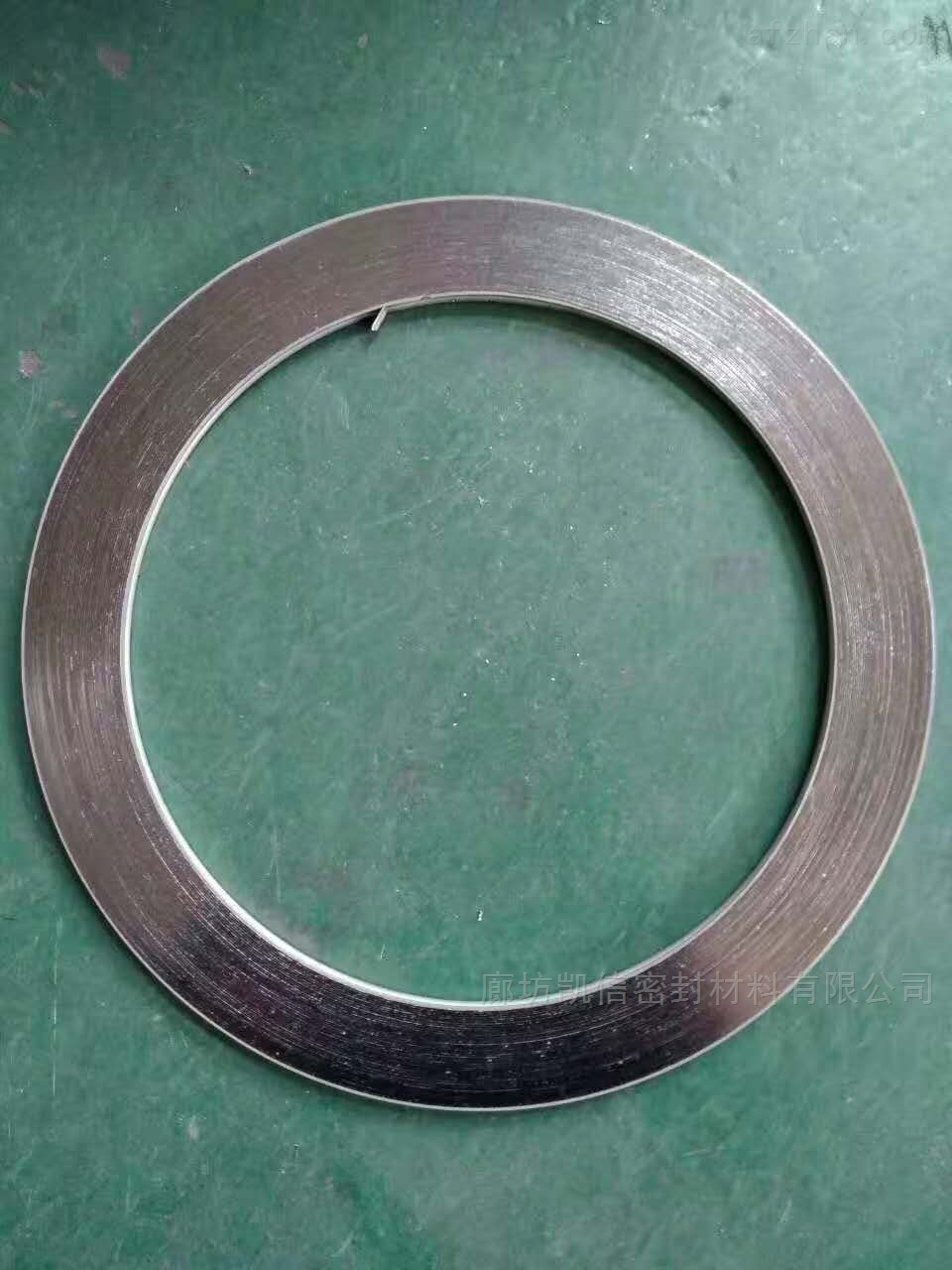DN50-150LB-C2220金属缠绕垫标准

• 产地
• 所在地
• 河北省
• 河北廊坊市

C2220金属缠绕垫标准C2220金属缠绕垫标准

SUS 304

SUS 316

SUS 304

SUS 304

SUS 316L

SUS 316

SUS 316

SUS 316L

SUS 316L

DIM(mm)ANSI B16.5BS1560(mm)Mss sp-44API605公差内环内径垫片内径<=600<=24">=26" and <=34"±0.5>600 and <=800>24" and <=34>=36" and <=60"±0.9>800 and <=1600>34" and <=60" 1.3="">1600>60" ±1.6垫片外径<=600<=24 0.9="">600 and <=160000>24" and <=60" 1.81600="">60" ±2.2外环外径 >1/2" and <=60 0-1.0="">600 and <=1000>24" and <=34" 0-1.8="">1000 and <=1600>34" and <=60 --="34"--">